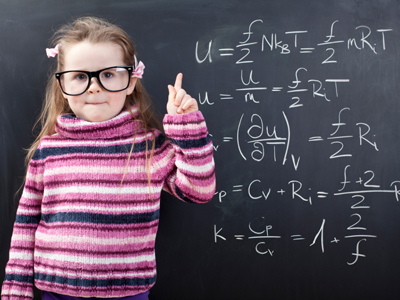Think before you answer each question.

# Algebra - Equations with Letters on Both Sides

This Math quiz is called 'Algebra - Equations with Letters on Both Sides' and it has been written by teachers to help you if you are studying the subject at middle school. Playing educational quizzes is a fabulous way to learn if you are in the 6th, 7th or 8th grade - aged 11 to 14.

It costs only \$12.50 per month to play this quiz and over 3,500 others that help you with your school work. You can subscribe on the page at Join Us

As is the case in Algebra, very often an equation will have letters on both sides of the equals sign. Remember the rule 'change sides, change signs' and you won't go very far wrong.

This Math quiz will give you plenty of practice.

1.
Look at the equation 4(a - 4) = 3a - 9. Now decide which of the following is not correct.
4a - 16 = 3a - 9
a = -9 + 16
a = 7
a = 25
2.
x + 7 = 10 + 12 is the same as which of the following?
x = 10 + 12 - 7
x = 10 + 12 + 7
x = 17 + 19
x = 70 + 84
When the 7 crosses over to the other side it becomes - 7
3.
What is the value of d in the following equation: 13d + 8 = 7d + 3d + 35?
7
9
11
13
'Write it down and work it out'. There is no alternative to a step by step approach!
4.
Look at this equation: 10x + 18x = 7x + 189. Now decide which of the following is not correct.
10x + 18x + 7x = 189
10x + 18x - 7x = 189
21x = 189
x = 9
5.
What is the value of e in the following equation: 2(e + 5) = 44?
0.7
7
17
27
6.
What is the value of c in the following equation: 4c = 2c + 75?
8
37.5
75
150
2c = 75
7.
4(a + 7) = 40 is the same as which of the following?
4 - a - 7 = 40
4 - a + 7 = 40
4 + a + 7 = 40
4a + 28 = 40
Multiply the 4 (outside of the brackets) with both the a and the 7 (inside the brackets)
8.
What is the value of f in the following equation: 7(f - 5) = 4(f - 3.5)?
7
9
11
13
9.
Look at this equation: 10 + 18 = 7x. Now decide which of the following is not correct.
7x = 10 + 18
7x = 10 - 18
7x = 28
x = 4
First get the letter x onto the left hand side and then, in stages, break down its value
10.
2x + 3y = 3y + 7 is the same as which of the following?
2x = 6y + 7
2x = 7
y = 2x - 7
y = 2x + 7
When + 3y crosses over to the other side it becomes - 3y and cancels out the 3y that was already on that side
Author:  Frank Evans